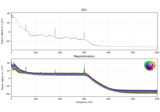# mne.compute_proj_evoked#

mne.compute_proj_evoked(evoked, n_grad=2, n_mag=2, n_eeg=2, desc_prefix=None, meg='separate', verbose=None)[source]#

Compute SSP (signal-space projection) vectors on evoked data.

This function aims to find those SSP vectors that will project out the `n` most prominent signals from the data for each specified sensor type. Consequently, if the provided input data contains high levels of noise, the produced SSP vectors can then be used to eliminate that noise from the data.

Parameters:
evokedinstance of `Evoked`

The Evoked obtained by averaging the artifact.

n_grad`int` | `float` between `0` and `1`

Number of vectors for gradiometers. Either an integer or a float between 0 and 1 to select the number of vectors to explain the cumulative variance greater than `n_grad`.

n_mag`int` | `float` between `0` and `1`

Number of vectors for magnetometers. Either an integer or a float between 0 and 1 to select the number of vectors to explain the cumulative variance greater than `n_mag`.

n_eeg`int` | `float` between `0` and `1`

Number of vectors for EEG channels. Either an integer or a float between 0 and 1 to select the number of vectors to explain the cumulative variance greater than `n_eeg`.

desc_prefix

The description prefix to use. If None, one will be created based on tmin and tmax.

New in v0.17.

meg`str`

Can be `'separate'` (default) or `'combined'` to compute projectors for magnetometers and gradiometers separately or jointly. If `'combined'`, `n_mag == n_grad` is required and the number of projectors computed for MEG will be `n_mag`.

New in v0.18.

verbose

Control verbosity of the logging output. If `None`, use the default verbosity level. See the logging documentation and `mne.verbose()` for details. Should only be passed as a keyword argument.

Returns:
projs

List of projection vectors.

## Examples using `mne.compute_proj_evoked`#Brainstorm raw (median nerve) dataset

Brainstorm raw (median nerve) dataset